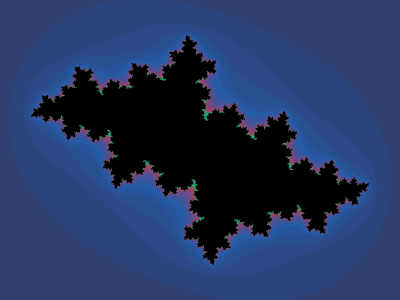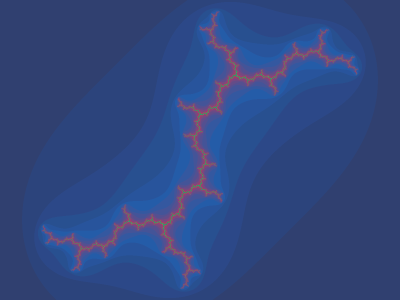#Julia Sets

Math Lair Home > Topics > Julia Sets

A Julia set is a type of fractal that, along with the Mandelbot set, has become an emblem of fractal geometry.

The Julia set is a somewhat difficult set to understand and is not easy to explain briefly. We'll start by looking at iterated functions over the set of real numbers. Start with the equation

xn+1 = f(xn) = xn²

What this equation is saying is that we'll start with a number, x1, square it, and the result will be x2, the next value that we'll use in the iteration. We'll then square x2 to get x3, and so on.

There are two possibilities as to what the result of the iteration would be. The first is that x will escape towards infinity. For example, if we were to select x1 = 2, the iterated values of x would be 4, 8, 16, 32, 64, ..., increasing without limit. The other possibility is that the result of the iteration will converge towards a value. If we were to select x1 = 0.5, the iteration would continue 0.25, 0.125, 0.0625, 0.03125, ..., approaching 0. The border between these two behaviours is, in this case, x = ±1.

We can iterate functions in the complex plane as well. For example, when we iterate zn+1 = f(zn) = zn² in the complex plane, we find that the boundary between the set of points that escape to infinity and those that converge to a finite value is a circle in the complex plane having radius 1. We could plot this in the complex plane. However, if we try other functions, such as f(z) = z² + c, where c is a parameter that a fairly small complex number, then the behaviour of the set is quite different, with the boundary exhibiting fractal behaviour. We call the set of points along the boundary the Julia set (often denoted J(f)), and the complement of that set (points not along the boundary) the Fatou set (often denoted F(f)). These sets are named after Gaston Julia and Pierre Fatou, who studied these sets in the early 20th century.

The beauty and complexity of Julia sets were not fully appreciated until fast computers with graphic capabilities were invented, which allowed Julia sets to be displayed visually.

The type of Julia set that is most often seen is that of f(z) = z² + c for a small complex value c.

One property Julia sets have are whether the values of the set are connected or disconnected. It can be shown (although the proof is much too involved to be presented here) that if f(0) converges, then the set is connected. If not, it is disconnected. The Mandelbrot set is the set of all values of c for which J is connected.

Here is a description of the behaviour of the Julia set for certain values of c:
Value of cDescription
(-0.1 + 0.1i)The set has an attractive fixed point, and J is a quasi-circle.
(-0.5 + 0.5i)The set has an attractive fixed point, and J is a quasi-circle.
(-1 + 0.05i)Attractive period-2 orbit.
(-0.2 + 0.75i)Attractive period-3 orbit.
(0.25 + 0.52i)Attractive period-4 orbit.
(-0.5 + 0.55i)Attractive period-5 orbit.
(0.66i)No attractive orbits and J is totally disconnected
(-i)J is a dendrite of tree-like form.

Here are some of these values plotted graphically. Note that, in these plots, the Julia set is the boundary between black areas (which converge) and coloured areas (which diverge).c = −0.5 + 0.5ic = 0.25 + 0.52ic = 0.66ic = −i